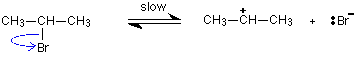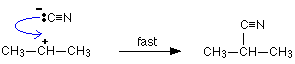Need help? Email help@shout.education

# Nucleophilic Substitution Reactions of Halogenoalkanes and Cyanide Ions

This page gives you the facts and simple, uncluttered mechanisms for the nucleophilic substitution reactions between halogenoalkanes and cyanide ions (from, for example, potassium cyanide). If you want the mechanisms explained to you in detail, there is a link at the bottom of the page.

## The Reaction of Primary Halogenoalkanes With Cyanide Ions

Important! If you aren't sure about the difference between primary, secondary and tertiary halogenoalkanes, it is essential that you follow this link before you go on.

### The Facts

If a halogenoalkane is heated under reflux with a solution of sodium or potassium cyanide in ethanol, the halogen is replaced by a -CN group and a nitrile is produced. Heating under reflux means heating with a condenser placed vertically in the flask to prevent loss of volatile substances from the mixture.

The solvent is important. If water is present you tend to get substitution by -OH instead of -CN.

Note: A solution of potassium cyanide in water is quite alkaline, and contains significant amounts of hydroxide ions. These react with the halogenoalkane.

For example, using 1-bromopropane as a typical primary halogenoalkane:

\text{CH}_3\text{CH}_2\text{CH}_2\text{Br} + \text{CN}^- \longrightarrow \text{CH}_3\text{CH}_2\text{CH}_2\text{CN} + \text{Br}^-

You could write the full equation rather than the ionic one, but it slightly obscures what's going on:

\text{CH}_3\text{CH}_2\text{CH}_2\text{Br} + \text{KCN} \longrightarrow \text{CH}_3\text{CH}_2\text{CH}_2\text{CN} + \text{KBr}

The bromine (or other halogen) in the halogenoalkane is simply replaced by a -CN group – hence a substitution reaction. In this example, butanenitrile is formed.

Note: When you are naming nitriles, you have to remember to include the carbon in the -CN group when you count the longest chain. In this example, there are 4 carbons in the longest chain – hence butanenitrile.

### The Mechanism

Here is the mechanism for the reaction involving bromoethane: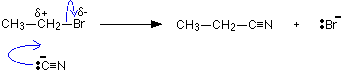This is an example of nucleophilic substitution.

Because the mechanism involves collision between two species in the slow step (in this case, the only step) of the reaction, it is known as an SN2 reaction.

Note: Unless your syllabus specifically mentions SN2 by name, you can just call it nucleophilic substitution.

If your examiners want you to show the transition state, draw the mechanism like this: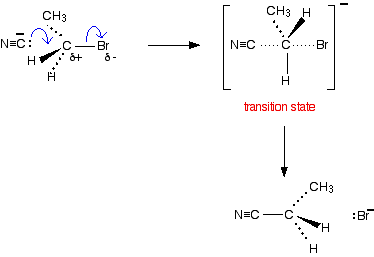## The Reaction of Tertiary Halogenoalkanes With Cyanide Ions

### The Facts

The facts of the reaction are exactly the same as with primary halogenoalkanes. If the halogenoalkane is heated under reflux with a solution of sodium or potassium cyanide in ethanol, the halogen is replaced by -CN, and a nitrile is produced.

For example:

(\text{CH}_3)_3\text{CBr} + \text{CN}^- \longrightarrow (\text{CH}_3)_3\text{CCN} + \text{Br}^-

Or if you want the full equation rather than the ionic one:

(\text{CH}_3)_3\text{CBr} + \text{KCN} \longrightarrow (\text{CH}_3)_3\text{CCN} + \text{KBr}

### The Mechanism

This mechanism involves an initial ionisation of the halogenoalkane: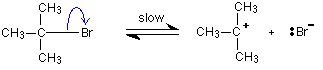followed by a very rapid attack by the cyanide ion on the carbocation (carbonium ion) formed: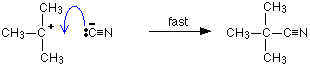This is again an example of nucleophilic substitution.

This time the slow step of the reaction only involves one species – the halogenoalkane. It is known as an SN1 reaction.

## The Reaction of Secondary Halogenoalkanes With Cyanide Ions

### The Facts

The facts of the reaction are exactly the same as with primary or tertiary halogenoalkanes. The halogenoalkane is heated under reflux with a solution of sodium or potassium cyanide in ethanol.

For example:

\text{CH}_3\text{CH}(\text{Br})\text{CH}_3 + \text{CN}^- \longrightarrow \text{CH}_3\text{CH}(\text{CN})\text{CH}_3 + \text{Br}^-

### The Mechanism

Secondary halogenoalkanes use both SN2 and SN1 mechanisms. For example, the SN2 mechanism is:

Should you need it, the two stages of the SN1 mechanism are: